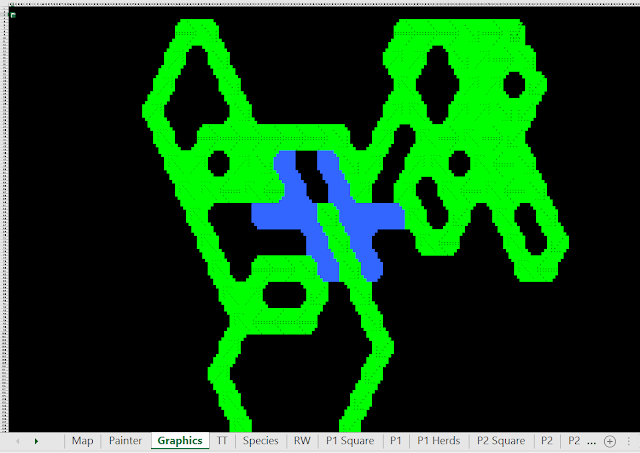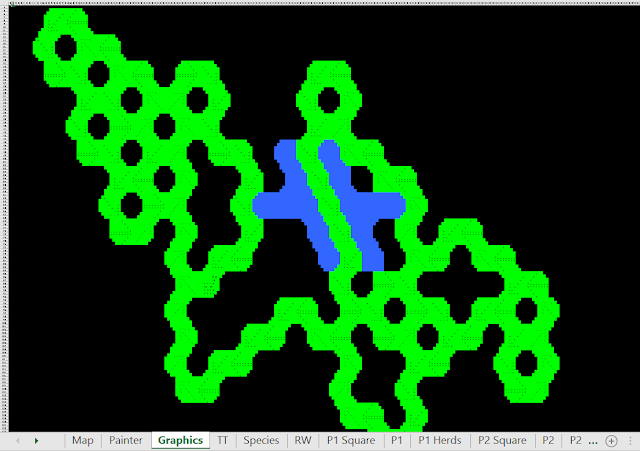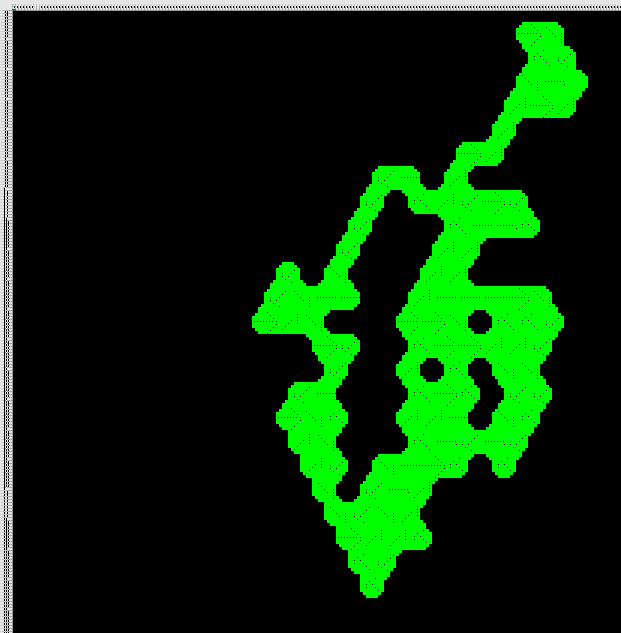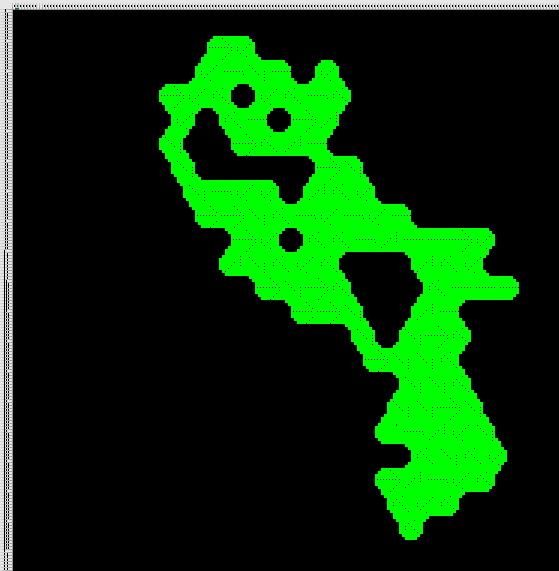## Friday, December 23, 2016

### Encyclopedia of Drunken Walks

"God does not play dice" vs "God does not waste computational resources"

Drunken walks are ideal for map generation for 3 reasons:

1. The generated map is inherently fractal.
2. The generated map is definitively random.
3. The map can be generated dynamically (on the fly).  This dynamic map generation allows for unlimited map size (boundless and infinite).

Drunken walks can be biased to favor any combination of the 6 cardinal/absolute directions (NW, NE, East, SE, SW, West) and the 6 relative directions (straight forward = 12:00, right forward = 2:00, right backward = 4:00, straight backward = 6:00, left backward = 8:00, left forward = 10:00).

These biases can be encoded in 6 weights for cardinal/absolute directions, and 6 weights for relative directions (relative to the current direction of movement).

A drunken walk pattern can thus be defined by 12 weights, and we call this collection of weights the "Direction Tensor".  The word "tensor" is used here to mean roughly "an object whose shadow is a vector".  At any given point in time, the likelihood of moving in each direction is proportional to the product of the cardinal/absolute weight, and the corresponding relative weight (each relative direction corresponds to an absolute direction, with respect to the current direction of movement).

The effects of chaging the 6 cardinal/absolute weights are fairly predictable, so this study examines only the effects of relative weightings (the 6 cardinal/absolute weights are taken to be equal to each other, so there are no cardinal biases).

- Example Patterns -

Limiting movement to backwards right and left (4:00 and 8:00) leads to a relatively compact but elongated form:50% right backwards (4:00), 50% left backwards (8:00).66% right backwards (4:00), 33% left backwards (8:00).
Limiting movement to straight and right forward (12:00 and 2:00) generates a less compact form:66% straight forward (12:00), 33% right forward (2:00).
Limiting motion to right forward and left forward (2:00 and 10:00) - aka clockwise and counter-clockwise - results in characteristic "holes".  This can be used for maps where regularly spaced lakes, islands, or oases are desired:50% right forward (2:00), 50% left forward (10:00).  [example 1/2]50% right forward (2:00), 50% left forward (10:00).  [example 2/2]
Allowing movement in any direction except for straight backwards leads to the following "balanced" typologies:20% straight forward (12:00), 20% right forward (2:00), 20% right backward (4:00), 20% left backward (8:00), 20% left forward (10:00).  [example 1/3]20% straight forward (12:00), 20% right forward (2:00), 20% right backward (4:00), 20% left backward (8:00), 20% left forward (10:00).  [example 2/3]20% straight forward (12:00), 20% right forward (2:00), 20% right backward (4:00), 20% left backward (8:00), 20% left forward (10:00).  [example 3/3]

- VBA Code (Class Module) -

'6 weights for the 6 absolute directions:
Public NW As Single
Public NE As Single
Public East As Single
Public SE As Single
Public SW As Single
Public West As Single

'6 weights for the 6 relative directions:
Public StraightForward As Single
Public RightForward As Single
Public RightBackward As Single
Public StraightBackward As Single
Public LeftBackward As Single
Public LeftForward As Single

'6 numbers for current fractional likelihood of each absolute direction:
Public ProbabilityNW As Single
Public ProbabilityNE As Single
Public ProbabilityEast As Single
Public ProbabilitySE As Single
Public ProbabilitySW As Single
Public ProbabilityWest As Single

Public Function GetDirection(ByVal Previous As Location, ByVal Current As Location)
Dim Direction As String
Dim SouthShift, EastShift As Integer

SouthShift = Current.Row - Previous.Row
EastShift = Current.Col - Previous.Col

Direction = "resting"
If SouthShift = 0 And EastShift = 1 Then Direction = "East"
If SouthShift = 0 And EastShift = -1 Then Direction = "West"
If SouthShift = 1 And EastShift = 0 Then Direction = "SE"
If SouthShift = 1 And EastShift = -1 Then Direction = "SW"
If SouthShift = -1 And EastShift = 1 Then Direction = "NE"
If SouthShift = -1 And EastShift = 0 Then Direction = "NW"

GetDirection = Direction
End Function

Public Sub CalculateProbabilities(ByVal Direction As String)
'The public variable "Direction" should be set before calling this sub.
Dim AbsoluteWeights(6) As Single
Dim RelativeWeights(6) As Single
Dim CombinedWeights(6) As Single
Dim Counter, RotatingCounter As Integer
Dim TotalWeights As Single

AbsoluteWeights(0) = Me.NW
AbsoluteWeights(1) = Me.NE
AbsoluteWeights(2) = Me.East
AbsoluteWeights(3) = Me.SE
AbsoluteWeights(4) = Me.SW
AbsoluteWeights(5) = Me.West

RelativeWeights(0) = Me.StraightForward
RelativeWeights(1) = Me.RightForward
RelativeWeights(2) = Me.RightBackward
RelativeWeights(3) = Me.StraightBackward
RelativeWeights(4) = Me.LeftBackward
RelativeWeights(5) = Me.LeftForward

TotalWeights = 0
'Rotate RelativeWeights depending on Direction:
Select Case Direction
Case "NW"
For Counter = 0 To 5
CombinedWeights(Counter) = AbsoluteWeights(Counter) * RelativeWeights(Counter)
TotalWeights = TotalWeights + CombinedWeights(Counter)
Next Counter
Case "NE"
For Counter = 0 To 5
RotatingCounter = (Counter - 1) Mod 6
'We need the next line, because vba does "mod" wrong.
If RotatingCounter < 0 Then RotatingCounter = RotatingCounter + 6
CombinedWeights(Counter) = AbsoluteWeights(Counter) * RelativeWeights(RotatingCounter)
TotalWeights = TotalWeights + CombinedWeights(Counter)
Next Counter
Case "East"
For Counter = 0 To 5
RotatingCounter = (Counter - 2) Mod 6
If RotatingCounter < 0 Then RotatingCounter = RotatingCounter + 6
CombinedWeights(Counter) = AbsoluteWeights(Counter) * RelativeWeights(RotatingCounter)
TotalWeights = TotalWeights + CombinedWeights(Counter)
Next Counter
Case "SE"
For Counter = 0 To 5
RotatingCounter = (Counter - 3) Mod 6
If RotatingCounter < 0 Then RotatingCounter = RotatingCounter + 6
CombinedWeights(Counter) = AbsoluteWeights(Counter) * RelativeWeights(RotatingCounter)
TotalWeights = TotalWeights + CombinedWeights(Counter)
Next Counter
Case "SW"
For Counter = 0 To 5
RotatingCounter = (Counter - 4) Mod 6
If RotatingCounter < 0 Then RotatingCounter = RotatingCounter + 6
CombinedWeights(Counter) = AbsoluteWeights(Counter) * RelativeWeights(RotatingCounter)
TotalWeights = TotalWeights + CombinedWeights(Counter)
Next Counter
Case "West"
For Counter = 0 To 5
RotatingCounter = (Counter - 5) Mod 6
If RotatingCounter < 0 Then RotatingCounter = RotatingCounter + 6
CombinedWeights(Counter) = AbsoluteWeights(Counter) * RelativeWeights(RotatingCounter)
TotalWeights = TotalWeights + CombinedWeights(Counter)
Next Counter
Case "resting"
'If the animal rested on the last turn, absolute/cardinal movement bias controls:
For Counter = 0 To 5
CombinedWeights(Counter) = AbsoluteWeights(Counter)
TotalWeights = TotalWeights + CombinedWeights(Counter)
Next Counter
End Select

'Normalize the probabilities so they add up to 1:
If TotalWeights <> 0 Then
For Counter = 0 To 5
CombinedWeights(Counter) = CombinedWeights(Counter) / TotalWeights
Next Counter
End If

Me.ProbabilityNW = CombinedWeights(0)
Me.ProbabilityNE = CombinedWeights(1)
Me.ProbabilityEast = CombinedWeights(2)
Me.ProbabilitySE = CombinedWeights(3)
Me.ProbabilitySW = CombinedWeights(4)
Me.ProbabilityWest = CombinedWeights(5)
End Sub

Public Sub NormalizeProbabilities(ByVal TotalWeights As Single)
'Use zero to indicate "recalculate TotalWeights":
If TotalWeights = 0 Then
TotalWeights = Me.ProbabilityNW + Me.ProbabilityNE + Me.ProbabilityEast _
+ Me.ProbabilitySE + Me.ProbabilitySW + Me.ProbabilityWest
End If

'If it actually is zero (based on math), then use "absolute values":
If TotalWeights = 0 Then
Me.CalculateProbabilities ("resting")
Else
Me.ProbabilityNW = Me.ProbabilityNW / TotalWeights
Me.ProbabilityNE = Me.ProbabilityNE / TotalWeights
Me.ProbabilityEast = Me.ProbabilityEast / TotalWeights
Me.ProbabilitySE = Me.ProbabilitySE / TotalWeights
Me.ProbabilitySW = Me.ProbabilitySW / TotalWeights
Me.ProbabilityWest = Me.ProbabilityWest / TotalWeights
End If
End Sub

```Copyright 2016 Matthew J Curran.